# FILLP

fillp = fill pattern

`` fillp( p )``
 `p` a number value (0-32768) that sets the pattern

`fillp(P)` is a PICO-8 lua function that sets a 4x4 2-color pattern that is tiled when drawing shape functions to the screen. Functions such as: `circ`, `circfill`, `rect`, `rectfill`, `oval`, `ovalfill`, `pset`, and `line`.

The 4x4 pixel grid used by `fillp` looks like this; with each pixel designated by a value: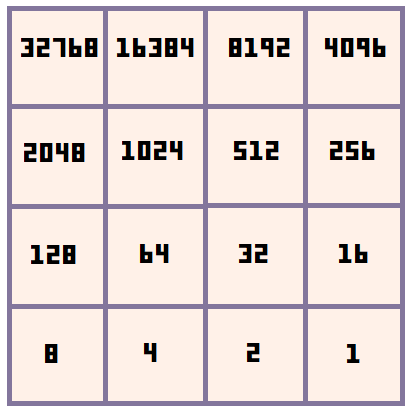The pattern set by `fillp()` is specified by the value of the argument ( `P` used here as an example ), which is a bitfield. The default value is 0, so `fillp()` or `fillp(0)` both reset the pattern to a full solid fill pattern. Then if we set the value to 1, then it will flip the bottom right pixel.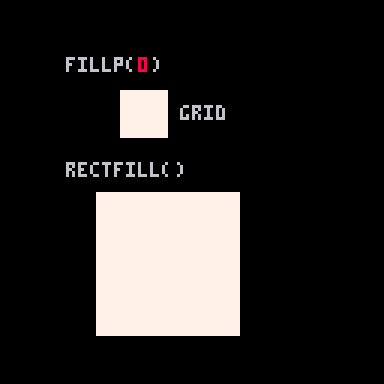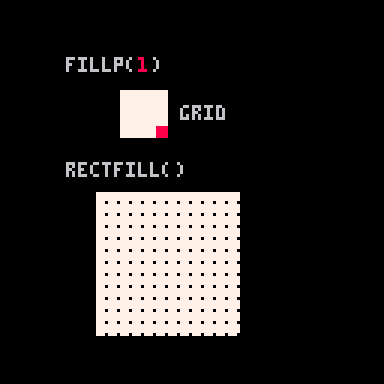If we continue adding just 1 to the value, then you'll see that a value of 2 will flip the second pixel on the bottom right, but not the bottom right corner anymore. Refer back to the grid above to understand how each pixel in the grid has a value.

Continuing on, with a value of 3, will flip both the first and second pixels on the bottom right because 3 is 1 and 2: ( 3 = 1 + 2 ).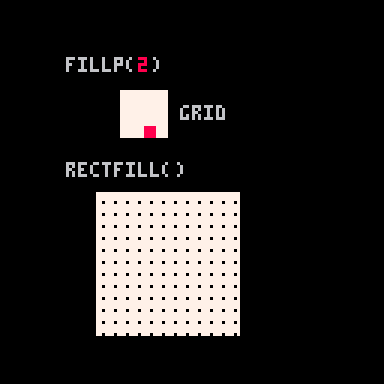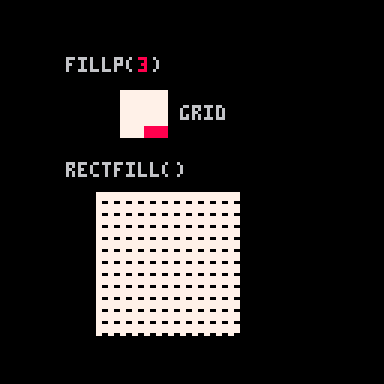Just like we added the bit values of 1 and 2 to get the pattern value of 3, you can find the exact pattern you want by simply added together the bit values. For example:

``fillp(2+8+16+64) --90``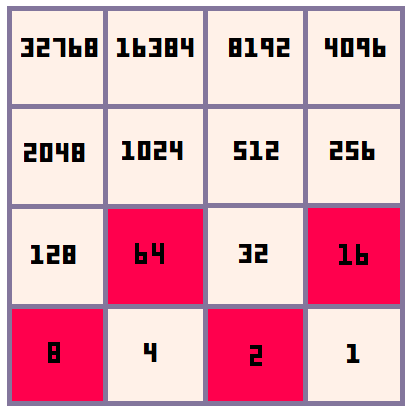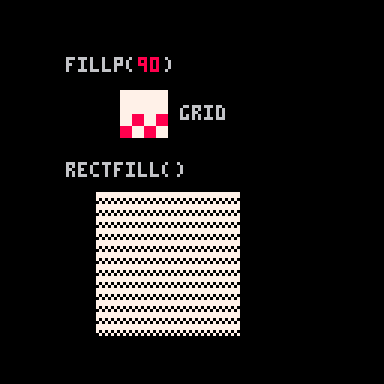Here are some more example fill patterns: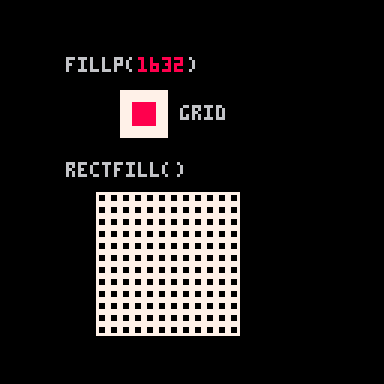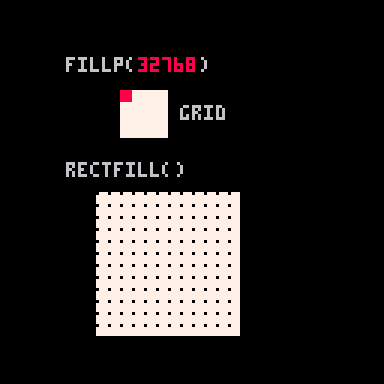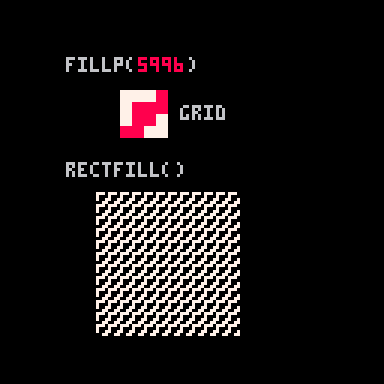For example, `fillp(4+8+64+128+256+512+4096+8192)` would produce a checkerboard pattern, which can also be expressed in binary as fillp(0b0011001111001100).

``````fillp(4+8+64+128+256+512+4096+8192)
fillp(0b0011001111001100)``````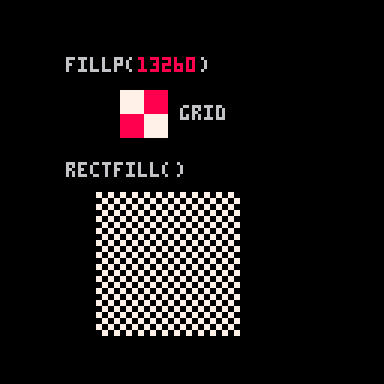To be added: Further explanation for setting a second color using "high bits", manual example: [ CIRCFILL(64,64,20, 0x4E) -- brown and pink ] and how to set fillp to apply transparency, or to sprites, and secondary palette. See the manual for more information.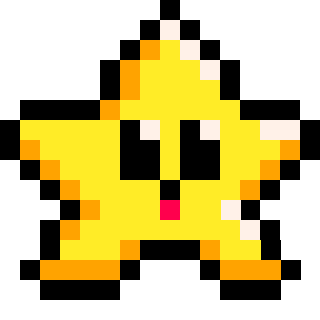104

21 Mar 2023

`Font`